Related Articles

# Python Plotly tutorial

• Last Updated : 04 Jun, 2021

Python Plotly Library is an open-source library that can be used for data visualization and understanding data simply and easily. Plotly supports various types of plots like line charts, scatter plots, histograms, cox plots, etc. So you all must be wondering why Plotly over other visualization tools or libraries? Here’s the answer –

• Plotly has hover tool capabilities that allow us to detect any outliers or anomalies in a large number of data points.
• It is visually attractive that can be accepted by a wide range of audiences.
• It allows us for the endless customization of our graphs that makes our plot more meaningful and understandable for others.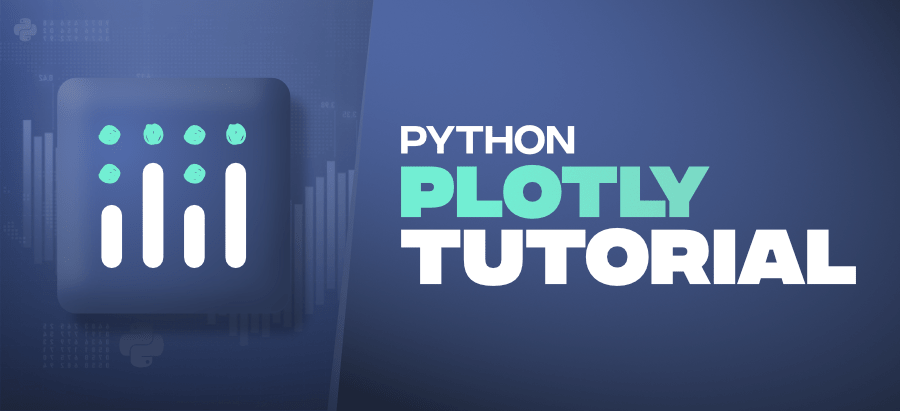This tutorial aims at providing you the insight about Plotly with the help of the huge dataset explaining the Plotly from basics to advance and covering all the popularly used charts.

Table Of Content

Recent Articles on Plotly !!!

## Installation

Plotly does not come built-in with Python. To install it type the below command in the terminal.

`pip install plotly`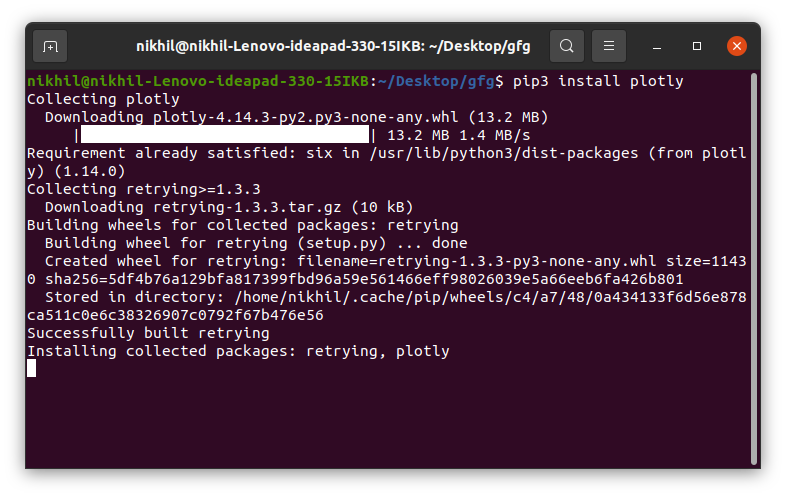This may take some time as it will install the dependencies as well.

## Package Structure of Plotly

There are three main modules in Plotly. They are:

• plotly.plotly
• plotly.graph.objects
• plotly.tools

plotly.plotly acts as the interface between the local machine and Plotly. It contains functions that require a response from Plotly’s server.

plotly.graph_objects module contains the objects (Figure, layout, data, and the definition of the plots like scatter plot, line chart) that are responsible for creating the plots.  The Figure can be represented either as dict or instances of plotly.graph_objects.Figure and these are serialized as JSON before it gets passed to plotly.js. Consider the below example for better understanding.

Note: plotly.express module can create the entire Figure at once. It uses the graph_objects internally and returns the graph_objects.Figure instance.

Example:

## Python3

 `import` `plotly.express as px `` ` ` ` `# Creating the Figure instance``fig ``=` `px.line(x``=``[``1``,``2``, ``3``], y``=``[``1``, ``2``, ``3``]) `` ` `# printing the figure instance``print``(fig)`

Output: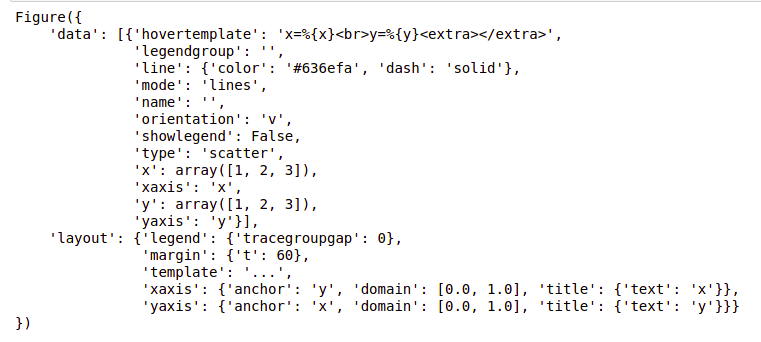Figures are represented as trees where the root node has three top layer attributes – data, layout, and frames and the named nodes called ‘attributes’. Consider the above example, layout.legend is a nested dictionary where the legend is the key inside the dictionary whose value is also a dictionary.

plotly.tools module contains various tools in the forms of the functions that can enhance the Plotly experience.

## Getting Started

After learning the installation and basic structure of the Plotly, let’s create a simple plot using the pre-defined data sets defined by the plotly.

Example:

## Python3

 `import` `plotly.express as px `` ` ` ` `# Creating the Figure instance``fig ``=` `px.line(x``=``[``1``, ``2``, ``3``], y``=``[``1``, ``2``, ``3``]) `` ` `# showing the plot``fig.show()`

Output: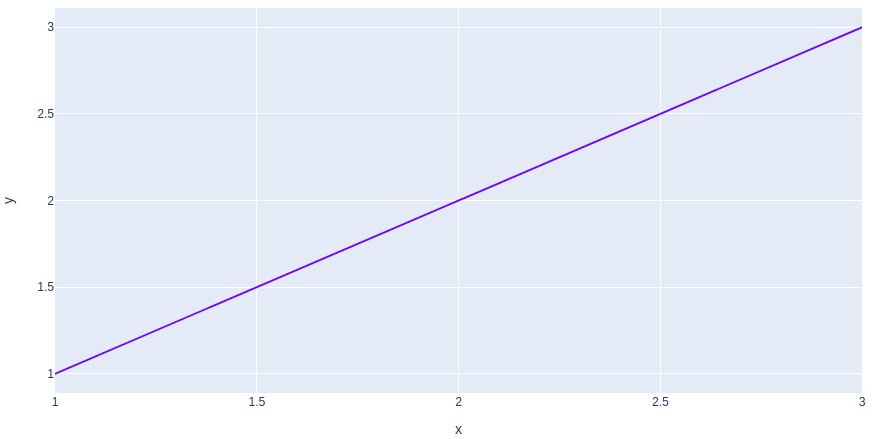In the above example, the plotly.express module is imported which returns the Figure instance. We have created a simple line chart by passing the x, y coordinates of the points to be plotted.

## Creating Different Types of Charts

With plotly we can create more than 40 charts and every plot can be created using the plotly.express and plotly.graph_objects class. Let’s see some commonly used charts with the help of Plotly.

### Line Chart

Line plot in Plotly is much accessible and illustrious annexation to plotly which manage a variety of types of data and assemble easy-to-style statistic. With px.line each data position is represented as a vertex  (which location is given by the x and y columns) of a polyline mark in 2D space.

Example:

## Python3

 `import` `plotly.express as px `` ` `# using the iris dataset``df ``=` `px.data.iris() `` ` `# plotting the line chart``fig ``=` `px.line(df, x``=``"species"``, y``=``"petal_width"``) `` ` `# showing the plot``fig.show()`

Output: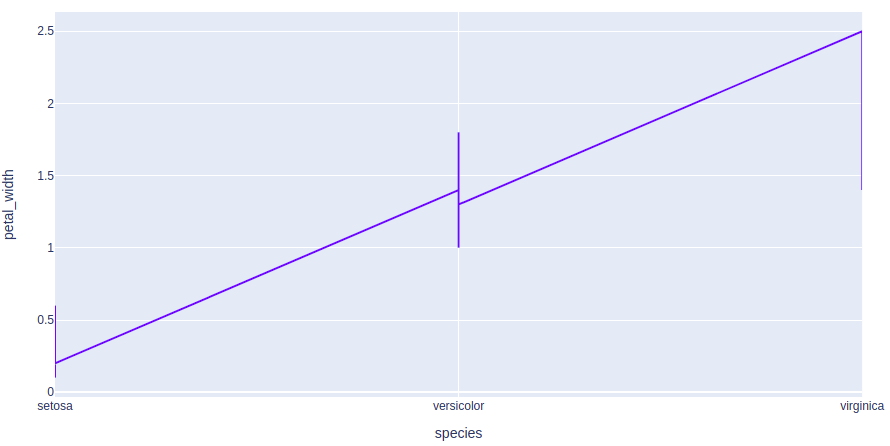Refer to the below articles to get detailed information about the line charts.

### Bar Chart

A bar chart is a pictorial representation of data that presents categorical data with rectangular bars with heights or lengths proportional to the values that they represent. In other words, it is the pictorial representation of dataset. These data sets contain the numerical values of variables that represent the length or height.

Example:

## Python3

 `import` `plotly.express as px `` ` `# using the iris dataset``df ``=` `px.data.iris() `` ` `# plotting the bar chart``fig ``=` `px.bar(df, x``=``"sepal_width"``, y``=``"sepal_length"``) `` ` `# showing the plot``fig.show()`

Output: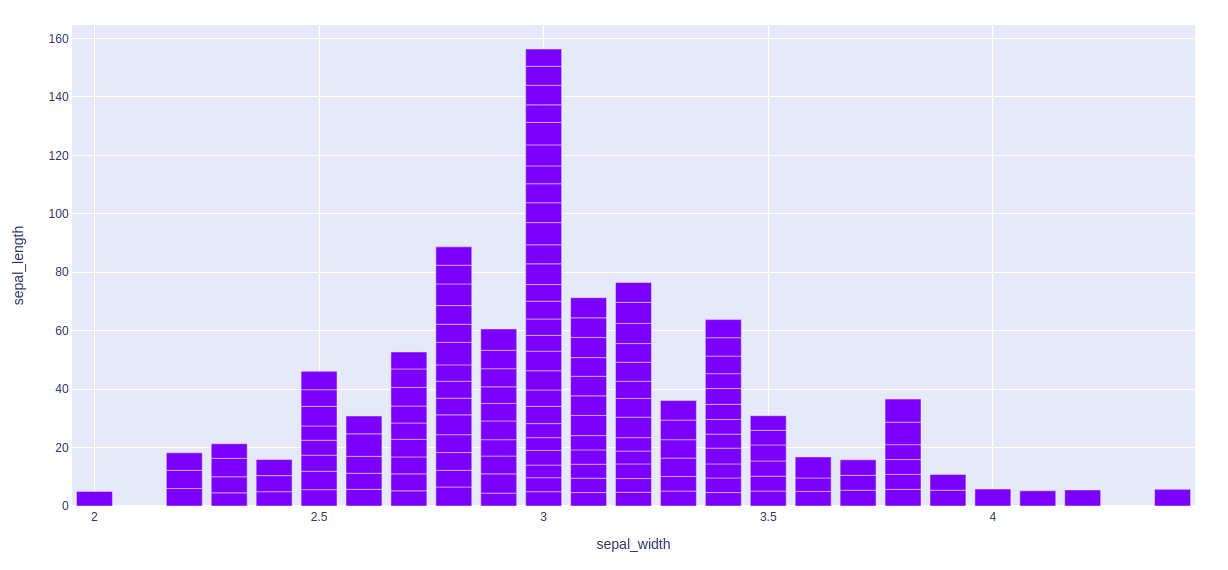Refer to the below articles to get detailed information about the bar chart.

### Histograms

A histogram contains a rectangular area to display the statistical information which is proportional to the frequency of a variable and its width in successive numerical intervals. A graphical representation that manages a group of data points into different specified ranges. It has a special feature that shows no gaps between the bars and similar to a vertical bar graph.

Example:

## Python3

 `import` `plotly.express as px `` ` `# using the iris dataset``df ``=` `px.data.iris() `` ` `# plotting the histogram``fig ``=` `px.histogram(df, x``=``"sepal_length"``, y``=``"petal_width"``) `` ` `# showing the plot``fig.show()`

Output: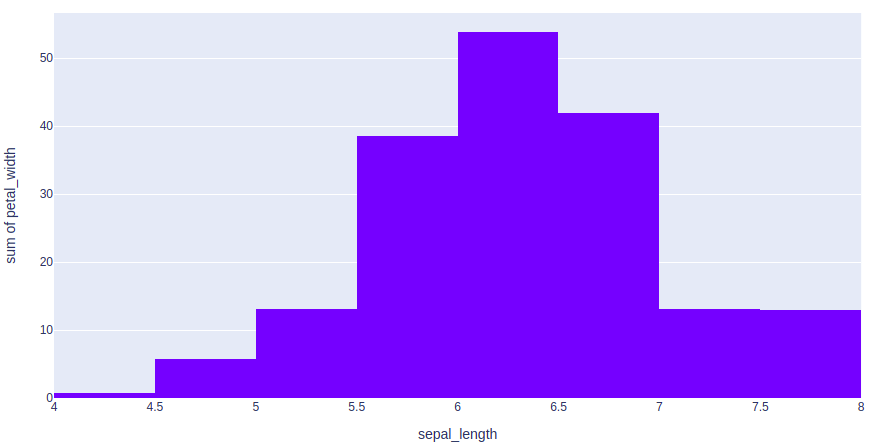Refer to the below articles to get detailed information about the histograms.

### Scatter Plot and Bubble charts

A scatter plot is a set of dotted points to represent individual pieces of data in the horizontal and vertical axis. A graph in which the values of two variables are plotted along X-axis and Y-axis, the pattern of the resulting points reveals a correlation between them.

A bubble plot is a scatter plot with bubbles (color-filled circles). Bubbles have various sizes dependent on another variable in the data. It can be created using the scatter() method of plotly.express.

Example 1: Scatter Plot

## Python3

 `import` `plotly.express as px `` ` `# using the iris dataset``df ``=` `px.data.iris() `` ` `# plotting the scatter chart``fig ``=` `px.scatter(df, x``=``"species"``, y``=``"petal_width"``) `` ` `# showing the plot``fig.show()`

Output: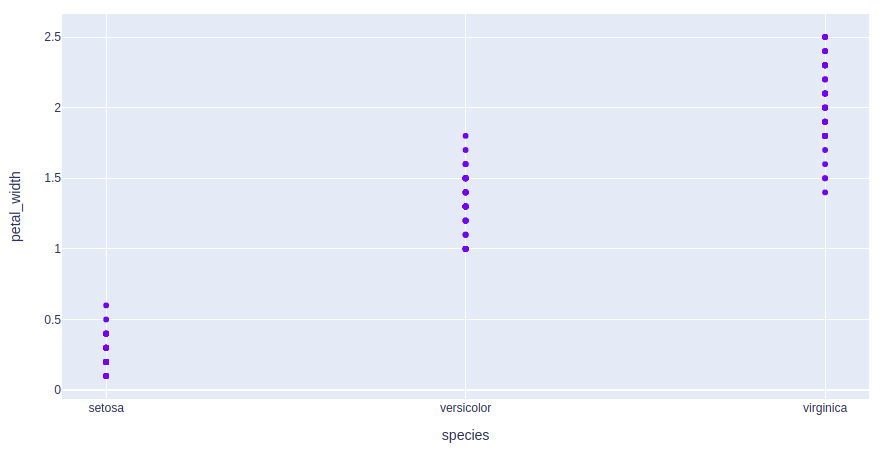Example 2: Bubble Plot

## Python3

 `import` `plotly.express as px `` ` `# using the iris dataset``df ``=` `px.data.iris() `` ` `# plotting the bubble chart``fig ``=` `px.scatter(df, x``=``"species"``, y``=``"petal_width"``, ``                 ``size``=``"petal_length"``, color``=``"species"``) `` ` `# showing the plot``fig.show()`

Output: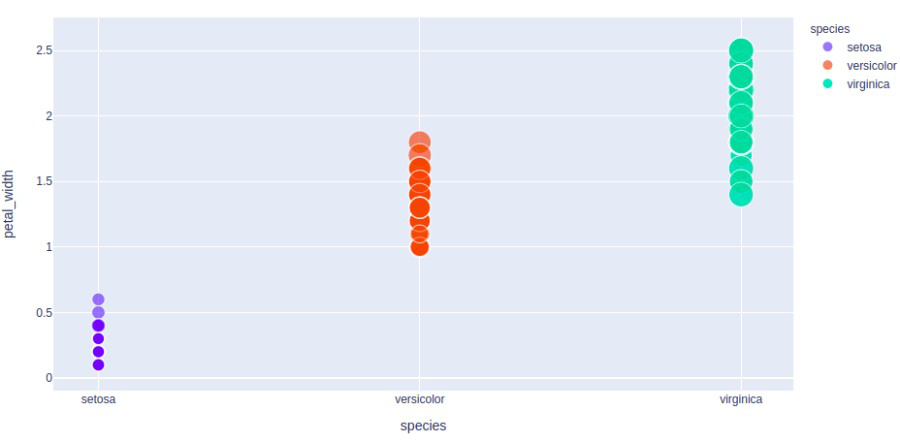Refer to the below articles to get detailed information about the scatter plots and bubble plots.

### Pie Charts

A pie chart is a circular statistical graphic, which is divided into slices to illustrate numerical proportions. It depicts a special chart that uses “pie slices”, where each sector shows the relative sizes of data. A circular chart cuts in a form of radii into segments describing relative frequencies or magnitude also known as circle graph.

Example:

## Python3

 `import` `plotly.express as px `` ` `# using the tips dataset``df ``=` `px.data.tips() `` ` `# plotting the pie chart``fig ``=` `px.pie(df, values``=``"total_bill"``, names``=``"day"``) `` ` `# showing the plot``fig.show()`

Output: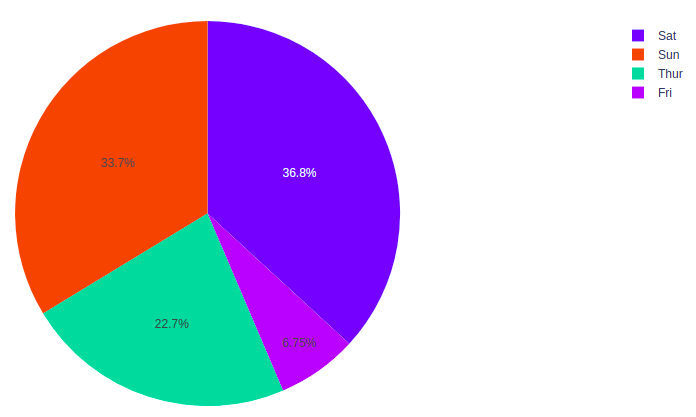Refer to the below articles to get detailed information about the pie charts.

### Box Plots

A Box Plot is also known as Whisker plot is created to display the summary of the set of data values having properties like minimum, first quartile, median, third quartile and maximum. In the box plot, a box is created from the first quartile to the third quartile, a verticle line is also there which goes through the box at the median. Here x-axis denotes the data to be plotted while the y-axis shows the frequency distribution.

Example:

## Python3

 `import` `plotly.express as px `` ` `# using the tips dataset``df ``=` `px.data.tips() `` ` `# plotting the box chart``fig ``=` `px.box(df, x``=``"day"``, y``=``"total_bill"``) `` ` `# showing the plot``fig.show()`

Output: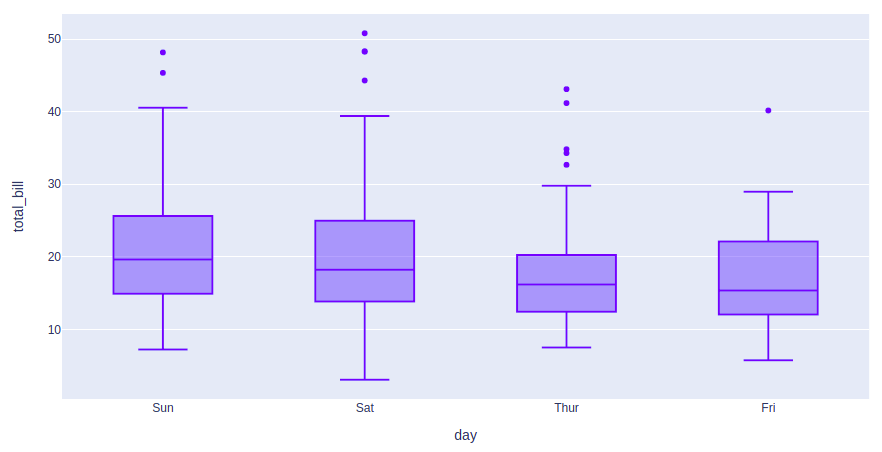Refer to the below articles to get detailed information about box plots.

### Violin plots

Violin Plot is a method to visualize the distribution of numerical data of different variables. It is similar to Box Plot but with a rotated plot on each side, giving more information about the density estimate on the y-axis. The density is mirrored and flipped over and the resulting shape is filled in, creating an image resembling a violin. The advantage of a violin plot is that it can show nuances in the distribution that aren’t perceptible in a boxplot. On the other hand, the boxplot more clearly shows the outliers in the data.

Example:

## Python3

 `import` `plotly.express as px `` ` `# using the tips dataset``df ``=` `px.data.tips() `` ` `# plotting the violin chart``fig ``=` `px.violin(df, x``=``"day"``, y``=``"total_bill"``)`` ` `# showing the plot``fig.show()`

Output: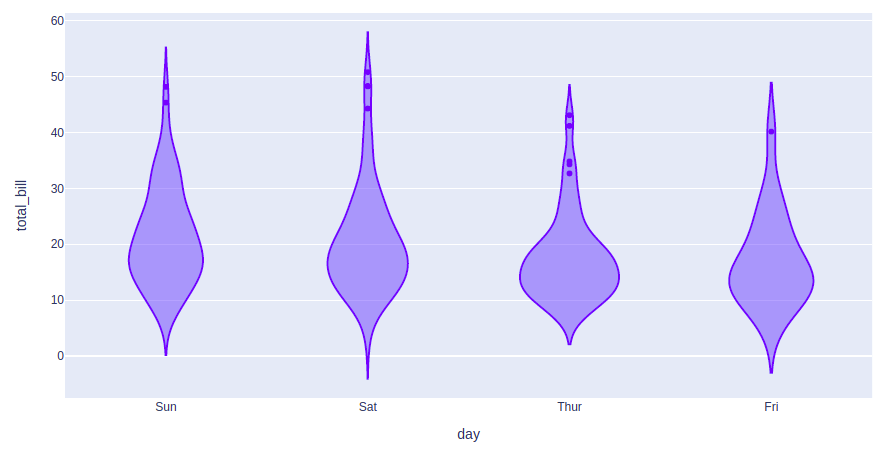Refer to the below articles to get detailed information about the violin plots.

• Violin Plots using Plotly

### Gantt Charts

Generalized Activity Normalization Time Table (GANTT) chart is type of chart in which series of horizontal lines are present that show the amount of work done or production completed in given period of time in relation to amount planned for those projects.

Example:

## Python3

 `import` `plotly.figure_factory as ff `` ` `# Data to be plotted``df ``=` `[``dict``(Task``=``"A"``, Start``=``'2020-01-01'``, Finish``=``'2009-02-02'``), ``    ``dict``(Task``=``"Job B"``, Start``=``'2020-03-01'``, Finish``=``'2020-11-11'``), ``    ``dict``(Task``=``"Job C"``, Start``=``'2020-08-06'``, Finish``=``'2020-09-21'``)] `` ` `# Creating the plot``fig ``=` `ff.create_gantt(df) ``fig.show()`

Output: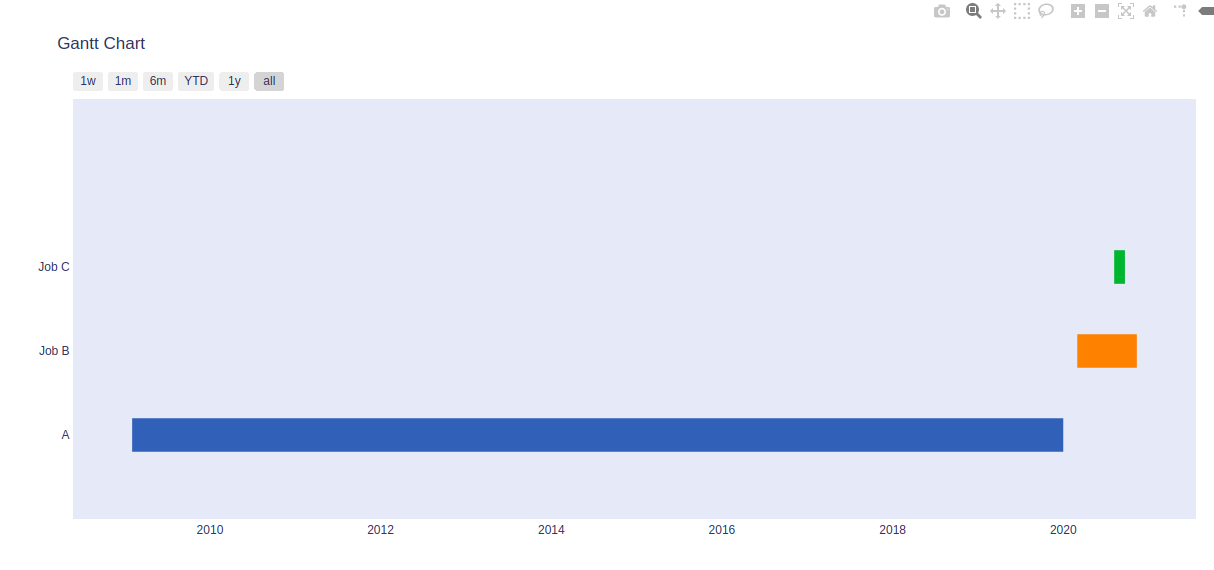Refer to the below articles to get detailed information about the Gantt Charts.

### Contour Plots

Contour plots also called level plots are a tool for doing multivariate analysis and visualizing 3-D plots in 2-D space. If we consider X and Y as our variables we want to plot then the response Z will be plotted as slices on the X-Y plane due to which contours are sometimes referred as Z-slices or iso-response.

A contour plots is used in the case where you want to see the changes in some value (Z) as a function with respect to the two values (X, Y). Consider the below example.

Example:

## Python3

 `import` `plotly.graph_objects as go `` ` ` ` `# Creating the X, Y value that will``# change the values of Z as a function``feature_x ``=` `np.arange(``0``, ``50``, ``2``) ``feature_y ``=` `np.arange(``0``, ``50``, ``3``) `` ` `# Creating 2-D grid of features ``[X, Y] ``=` `np.meshgrid(feature_x, feature_y) `` ` `Z ``=` `np.cos(X ``/` `2``) ``+` `np.sin(Y ``/` `4``) `` ` `# plotting the figure``fig ``=` `go.Figure(data ``=``    ``go.Contour(x ``=` `feature_x, y ``=` `feature_y, z ``=` `Z)) `` ` `fig.show()`

Output: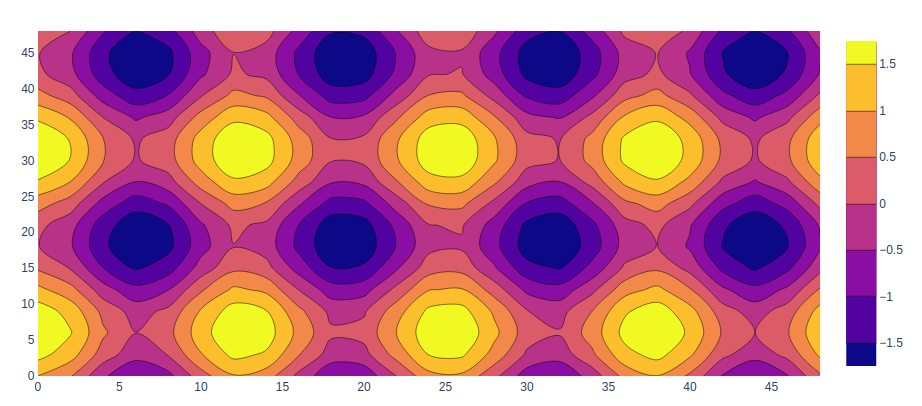Refer to the below articles to get detailed information about contour plots.

### Heatmaps

Heatmap is defined as a graphical representation of data using colors to visualize the value of the matrix. In this, to represent more common values or higher activities brighter colors basically reddish colors are used and to represent less common or activity values, darker colors are preferred. Heatmap is also defined by the name of the shading matrix.

Example:

## Python3

 `import` `plotly.graph_objects as go `` ` ` ` `feature_x ``=` `np.arange(``0``, ``50``, ``2``) ``feature_y ``=` `np.arange(``0``, ``50``, ``3``) `` ` `# Creating 2-D grid of features ``[X, Y] ``=` `np.meshgrid(feature_x, feature_y) `` ` `Z ``=` `np.cos(X ``/` `2``) ``+` `np.sin(Y ``/` `4``) `` ` `# plotting the figure``fig ``=` `go.Figure(data ``=``     ``go.Heatmap(x ``=` `feature_x, y ``=` `feature_y, z ``=` `Z,)) `` ` `fig.show()`

Output: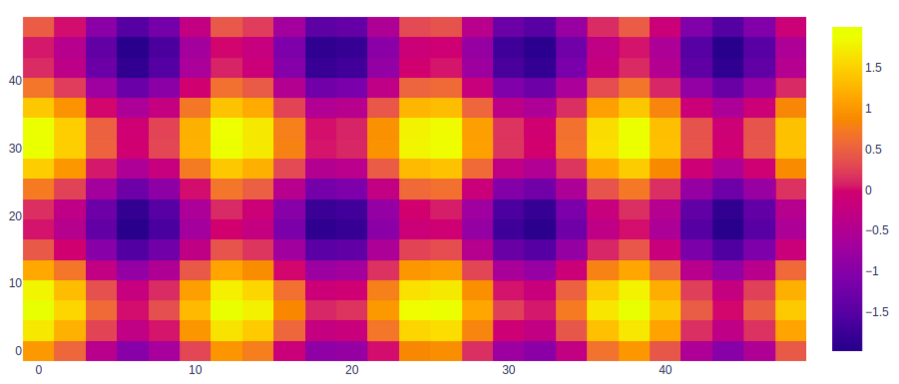Refer to the below articles to get detailed nformation about the heatmaps.

### Error Bars

For functions representing 2D data points such as px.scatter, px.line, px.bar, etc., error bars are given as a column name which is the value of the error_x (for the error on x position) and error_y (for the error on y position). Error bars are the graphical presentation alternation of data and used on graphs to imply the error or uncertainty in a reported capacity.

Example:

## Python3

 `import` `plotly.express as px `` ` `# using the iris dataset``df ``=` `px.data.iris() `` ` `# Calculating the error field``df[``"error"``] ``=` `df[``"petal_length"``]``/``100`` ` `# plotting the scatter chart``fig ``=` `px.scatter(df, x``=``"species"``, y``=``"petal_width"``,``                ``error_x``=``"error"``, error_y``=``"error"``) `` ` `# showing the plot``fig.show()`

Output: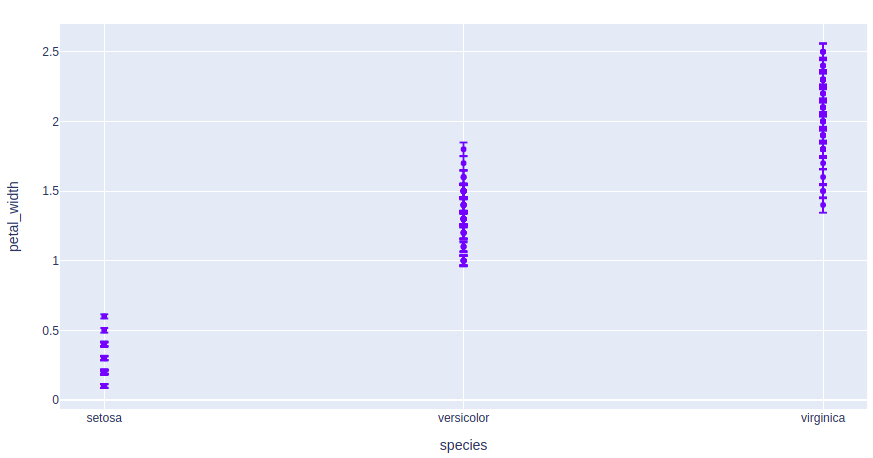### 3D Line Plots

Line plot in plotly is much accessible and illustrious annexation to plotly which manage a variety of types of data and assemble easy-to-style statistic. With px.line_3d each data position is represented as a vertex  (which location is given by the x, y and z columns) of a polyline mark in 3D space.

Example:

## Python3

 `import` `plotly.express as px `` ` `# data to be pltted``df ``=` `px.data.tips() `` ` `# plotting the figure``fig ``=` `px.line_3d(df, x``=``"sex"``, y``=``"day"``, ``                 ``z``=``"time"``, color``=``"sex"``) `` ` `fig.show()`

Output: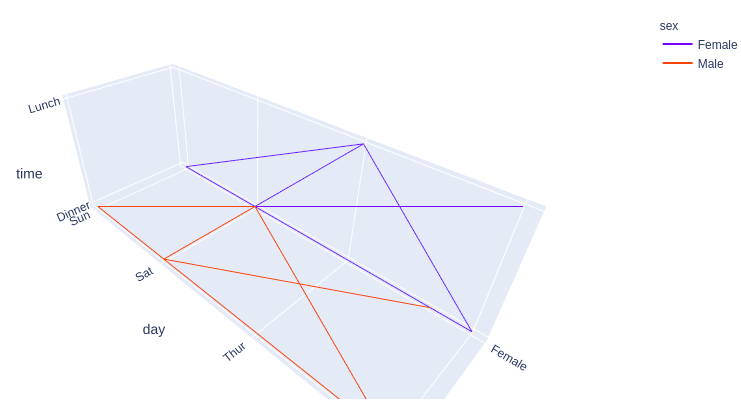Refer to the below articles to get detailed information about the 3D line charts.

### 3D Scatter Plot Plotly

3D Scatter Plot can plot two-dimensional graphics that can be enhanced by mapping up to three additional variables while using the semantics of hue, size, and style parameters. All the parameter control visual semantic which are used to identify the different subsets. Using redundant semantics can be helpful for making graphics more accessible. It can be created using the scatter_3d function of plotly.express class.

Example:

## Python3

 `import` `plotly.express as px `` ` `# Data to be plotted``df ``=` `px.data.iris() `` ` `# Plotting the figure``fig ``=` `px.scatter_3d(df, x ``=` `'sepal_width'``, ``                    ``y ``=` `'sepal_length'``, ``                    ``z ``=` `'petal_width'``, ``                    ``color ``=` `'species'``) `` ` `fig.show()`

Output: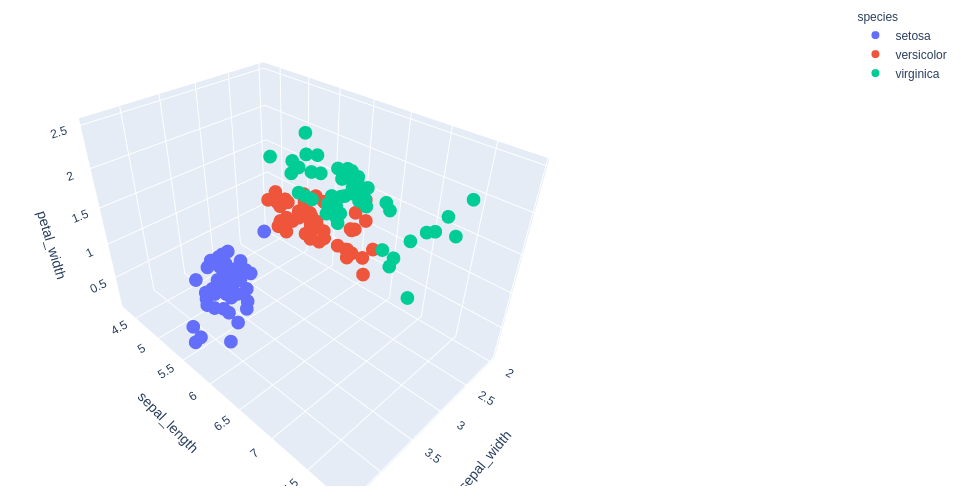Refer to the below articles to get detailed information about the 3D scatter plot.

### 3D Surface Plots

Surface plot is those plot which has three-dimensions data which is X, Y, and Z. Rather than showing individual data points, the surface plot has a functional relationship between dependent variable Y and have two independent variables X and Z. This plot is used to distinguish between dependent and independent variables.

Example:

## Python3

 `import` `plotly.graph_objects as go ``import` `numpy as np `` ` `# Data to be plotted``x ``=` `np.outer(np.linspace(``-``2``, ``2``, ``30``), np.ones(``30``)) ``y ``=` `x.copy().T ``z ``=` `np.cos(x ``*``*` `2` `+` `y ``*``*` `2``) `` ` `# plotting the figure``fig ``=` `go.Figure(data``=``[go.Surface(x``=``x, y``=``y, z``=``z)]) `` ` `fig.show()`

Output: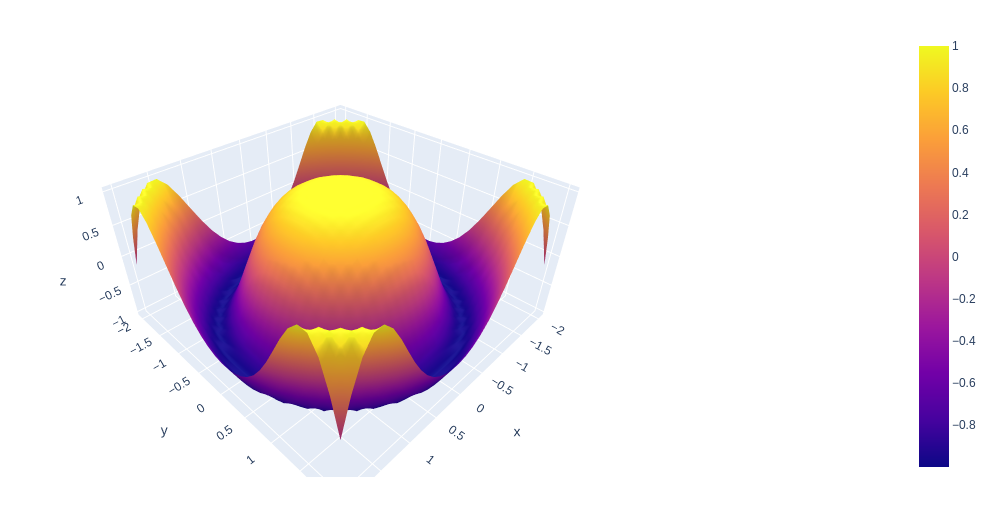## Interacting with the Plots

Plotly provides various tools for interacting with the plots such as adding dropdowns, buttons, sliders, etc. These can be created using the upatemenu attribute of the plot layout. Let’s see how to do all such things in detail.

A drop-down menu is a part of the menu-button which is displayed on a screen all the time. Every menu button is associated with a Menu widget that can display the choices for that menu button when clicked on it. In plotly, there are 4 possible methods to modify the charts by using updatemenu method.

• restyle: modify data or data attributes
• relayout: modify layout attributes
• update: modify data and layout attributes
• animate: start or pause an animation

Example:

## Python3

 `import` `plotly.graph_objects as px``import` `numpy as np`` ` ` ` `# creating random data through randomint``# function of numpy.random``np.random.seed(``42``)`` ` `# Data to be Plotted``random_x ``=` `np.random.randint(``1``, ``101``, ``100``)``random_y ``=` `np.random.randint(``1``, ``101``, ``100``)`` ` `plot ``=` `px.Figure(data``=``[px.Scatter(``    ``x``=``random_x,``    ``y``=``random_y,``    ``mode``=``'markers'``,)``])`` ` `# Add dropdown``plot.update_layout(``    ``updatemenus``=``[``        ``dict``(``            ``buttons``=``list``([``                ``dict``(``                    ``args``=``[``"type"``, ``"scatter"``],``                    ``label``=``"Scatter Plot"``,``                    ``method``=``"restyle"``                ``),``                ``dict``(``                    ``args``=``[``"type"``, ``"bar"``],``                    ``label``=``"Bar Chart"``,``                    ``method``=``"restyle"``                ``)``            ``]),``            ``direction``=``"down"``,``        ``),``    ``]``)`` ` `plot.show()`

Output:

In the above example we have created two graphs for the same data. These plots are accessible using the dropdown menu.

### Adding Buttons to the Plot

In plotly, actions custom Buttons are used to quickly make actions directly from a record. Custom Buttons can be added to page layouts in CRM, Marketing, and Custom Apps. There are also 4 possible methods that can be applied in custom buttons:

• restyle: modify data or data attributes
• relayout: modify layout attributes
• update: modify data and layout attributes
• animate: start or pause an animation

Example:

## Python3

 `import` `plotly.graph_objects as px``import` `pandas as pd`` ` `# reading the database``data ``=` `pd.read_csv(``"tips.csv"``)`` ` ` ` `plot ``=` `px.Figure(data``=``[px.Scatter(``    ``x``=``data[``'day'``],``    ``y``=``data[``'tip'``],``    ``mode``=``'markers'``,)``])`` ` `# Add dropdown``plot.update_layout(``    ``updatemenus``=``[``        ``dict``(``            ``type``=``"buttons"``,``            ``direction``=``"left"``,``            ``buttons``=``list``([``                ``dict``(``                    ``args``=``[``"type"``, ``"scatter"``],``                    ``label``=``"Scatter Plot"``,``                    ``method``=``"restyle"``                ``),``                ``dict``(``                    ``args``=``[``"type"``, ``"bar"``],``                    ``label``=``"Bar Chart"``,``                    ``method``=``"restyle"``                ``)``            ``]),``        ``),``    ``]``)`` ` `plot.show()`

Output: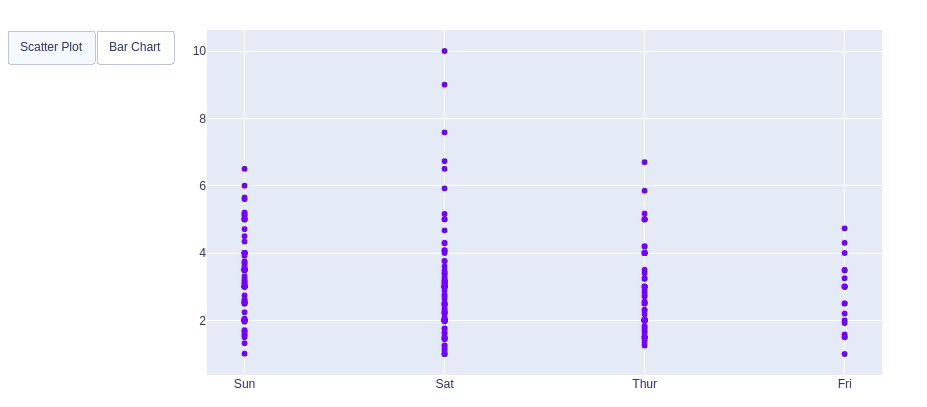In this example also we are creating two different plots on the same data and both plots are accessible by the buttons.

### Creating Sliders and Selectors to the Plot

In plotly, the range slider is a custom range-type input control. It allows selecting a value or a range of values between a specified minimum and maximum range. And the range selector is a tool for selecting ranges to display within the chart. It provides buttons to select pre-configured ranges in the chart. It also provides input boxes where the minimum and maximum dates can be manually input.

Example:

## Python3

 `import` `plotly.graph_objects as px ``import` `plotly.express as go ``import` `numpy as np `` ` `df ``=` `go.data.tips() `` ` `x ``=` `df[``'total_bill'``] ``y ``=` `df[``'day'``] `` ` `plot ``=` `px.Figure(data``=``[px.Scatter( ``    ``x``=``x, ``    ``y``=``y, ``    ``mode``=``'lines'``,) ``]) `` ` `plot.update_layout( ``    ``xaxis``=``dict``( ``        ``rangeselector``=``dict``( ``            ``buttons``=``list``([ ``                ``dict``(count``=``1``, ``                    ``step``=``"day"``, ``                    ``stepmode``=``"backward"``), ``            ``]) ``        ``), ``        ``rangeslider``=``dict``( ``            ``visible``=``True``        ``), ``    ``) ``) `` ` `plot.show() `

Output:

## More Topics on Plotly

Attention geek! Strengthen your foundations with the Python Programming Foundation Course and learn the basics.

To begin with, your interview preparations Enhance your Data Structures concepts with the Python DS Course. And to begin with your Machine Learning Journey, join the Machine Learning – Basic Level Course

My Personal Notes arrow_drop_up